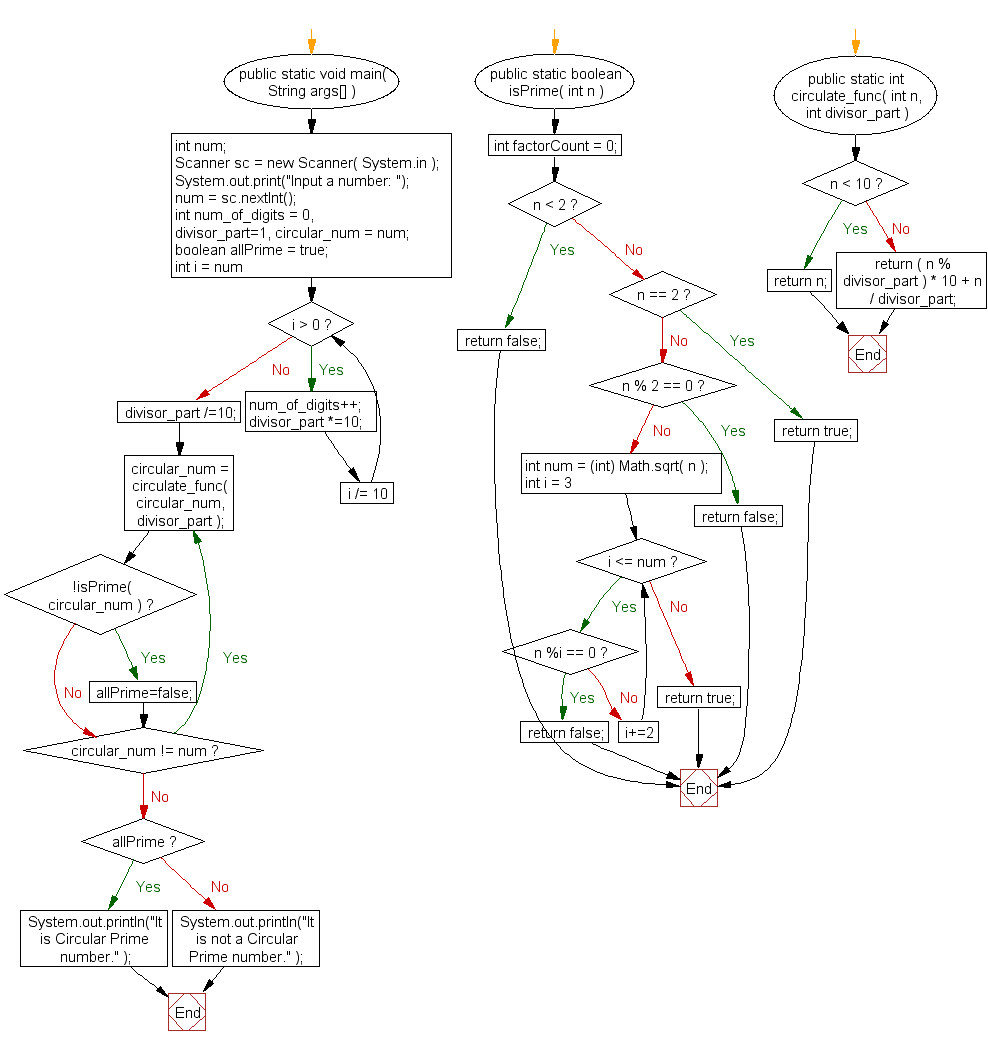﻿ Java exercises: Check if a given number is circular prime or not - w3resource# Java Exercises: Check if a given number is circular prime or not

## Java Numbers: Exercise-17 with Solution

Write a Java program to check if a given number is circular prime or not.

Circular Prime : A circular prime is a prime number with the property that the number generated at each intermediate step when cyclically permuting its (base 10) digits will be prime.

For example, 1193 is a circular prime, since 1931, 9311 and 3119 all are also prime. A circular prime with at least two digits can only consist of combinations of the digits 1, 3, 7 or 9, because having 0, 2, 4, 6 or 8 as the last digit makes the number divisible by 2, and having 0 or 5 as the last digit makes it divisible by 5.

Test Data
Input a number: 35

Pictorial Presentation:Sample Solution:

Java Code:

``````import java.util.Scanner;
public class Example17  {

public static void main( String args[] ){
int num;
Scanner sc = new Scanner( System.in );
System.out.print("Input a number: ");
num = sc.nextInt();
int num_of_digits = 0, divisor_part=1, circular_num = num;
boolean allPrime = true;
for( int i = num; i > 0; i /= 10 ){
num_of_digits++;
divisor_part *=10;
}
divisor_part /=10;
do{
circular_num = circulate_func( circular_num, divisor_part );
if( !isPrime( circular_num ) ) allPrime=false;
}while( circular_num != num );
if( allPrime ) System.out.println("It is Circular Prime number." );
else System.out.println("It is not a Circular Prime number." );
}

public static boolean isPrime( int n ){
int factorCount = 0;
if( n < 2 ) return false;
else if( n == 2 ) return true;
else if( n % 2 == 0 ) return false;
else{
int num = (int) Math.sqrt( n );
for( int i = 3 ; i <= num; i+=2 ){
if( n %i == 0 ){
return false;
}
}
}
return true;
}
public static int circulate_func( int n, int divisor_part ){
if( n < 10 ) return n;
else return ( n % divisor_part ) * 10 + n / divisor_part;
}

}
```
```

Sample Output:

```Input a number: 35
It is not a Circular Prime number.
```

Flowchart:Java Code Editor:

What is the difficulty level of this exercise?

﻿

## Java: Tips of the Day

Array vs ArrayLists:

The main difference between these two is that an Array is of fixed size so once you have created an Array you cannot change it but the ArrayList is not of fixed size. You can create instances of ArrayLists without specifying its size. So if you create such instances of an ArrayList without specifying its size Java will create an instance of an ArrayList of default size.

Once an ArrayList is full it re-sizes itself. In fact, an ArrayList is internally supported by an array. So when an ArrayList is resized it will slow down its performance a bit as the contents of the old Array must be copied to a new Array.

At the same time, it's compulsory to specify the size of an Array directly or indirectly while creating it. And also Arrays can store both primitives and objects while ArrayLists only can store objects.

Ref: https://bit.ly/3o8L2KH